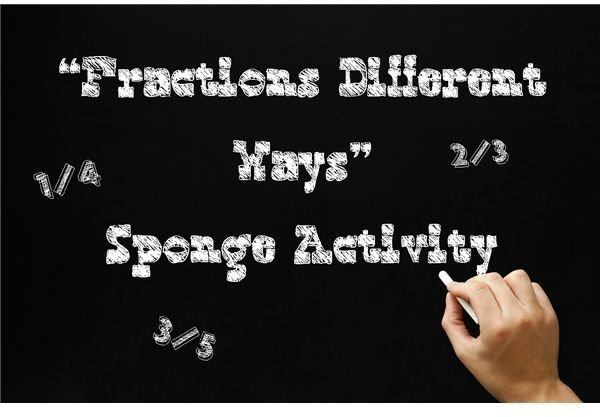Middle School Math Fractions Sponge ActivityObjective

Students will demonstrate understanding of fractions by showing different ways to express them.

Materials

• Reference material (pictures, displays, manipulatives, or other items preferred by teacher)
• Pencils
• Paper
• Timer

Note: students should be comfortable expressing fractions different ways, whether by showing them as the sum of a group of fractions, or as decimals and percentages to feel comfortable with this activity.

Lesson Procedure

Step One: Introduce a reference item, such as a color picture with many different objects in it or a tray with different items that can be compared using fractions. Ask students to identify fractions in the reference item (should limit the questions to a total of five for this activity).

For example, if the picture is of a group of animals, you might ask, “What fraction of the animals in the picture has fur? What fraction has feathers?”

If the reference item is a tray of desk supplies, you might ask, “What fraction of the items can be used to write? What fraction of the items has printed words on them?”

Step Two: Students should write down the fractions that answer the questions. Explain that students will get three minutes to express the fractions they have written down in as many different formats as possible, such as sums of smaller fractions, equivalent fractions, simplified fractions, decimals and percentages.

Start the timer and give students a few minutes to write down the different ways they can think of to express the fractions.

Step Three: Give students the opportunity to share their answers. If at the end of class, students might be dismissed upon sharing their answers. You might also choose to reward participation points for correct answers or use a process of elimination to determine the most unique fraction expressions and give a tangible reward to those students who came up with ways to express the fractions not used by their classmates.

Assessment

You may want to use this activity as an informal way to assess individual students’ abilities with expressing fractions, either by observation of collecting the written work of students.

Extension

Expand this activity by including ratios, asking students to write multiple ratio expressions of the objects they see in the provided reference materials!